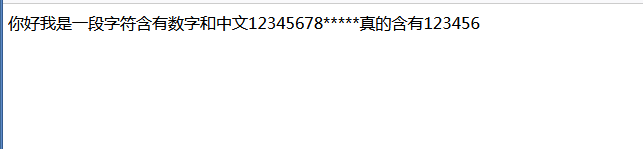2021-10-25 22:51

# asp隐藏这个字段中，有文字、有数字，如果有数字，超过10为数字的后5位数隐藏

asp隐藏这个字段中，有文字有数字，如果有数字，超过10为数字的后5位数隐藏。

<%
function hide(str)
str = left(str,len(str)-5) + "* * * * *"
hide = str
end function
%>

<%=hide(rsnews("这个字段名称"))%>

• 好问题 提建议
• 收藏

#### 3条回答默认 最新

•月小升2016 2021-10-26 14:20
``````
Function hide(strng)  '以数组返回
i = 0
Set regEx = New RegExp
regEx.Pattern = "(d+)" '"[0-9]"
regEx.IgnoreCase = True
regEx.Global = True
Set Matches = regEx.Execute(strng)
For Each Match in Matches
'RetStr = RetStr &"<br>"& Match.Value
If  Len(Match.Value)>=10 Then
strng = Replace(strng, Right(Match.Value,5),"*****")
End If
i = i + 1
Next
hide = strng
End Function
``````

核心思想是要用正则表达式来读取字符串里的数字

评论
解决 无用
打赏 举报
•王大师王文峰 2021-10-26 14:50

如果是asp可以用 HiddenField隐藏控件

评论
解决 无用
打赏 举报
•一把编程的菜刀 2021-10-26 15:17
``````Function RegExpTest(str)
Set regEx = New RegExp
regEx.Pattern = "(\d+)"
regEx.IgnoreCase = True
regEx.Global = True
Set Matches = regEx.Execute(str)
For Each Match in Matches
If  Len(Match.Value)>=10 Then
str= Replace(str, Right(Match.Value,5),"*****")
End If
Next
RegExpTest = str
End Function
str="你好我是一段字符含有数字和中文1234567890123真的含有123456"
response.write RegExpTest(str)

``````

asp 使用正则提取字符串中的数字，然后判断长度，替换，如有帮助，请点本答案给个采纳评论
解决 无用
打赏 举报